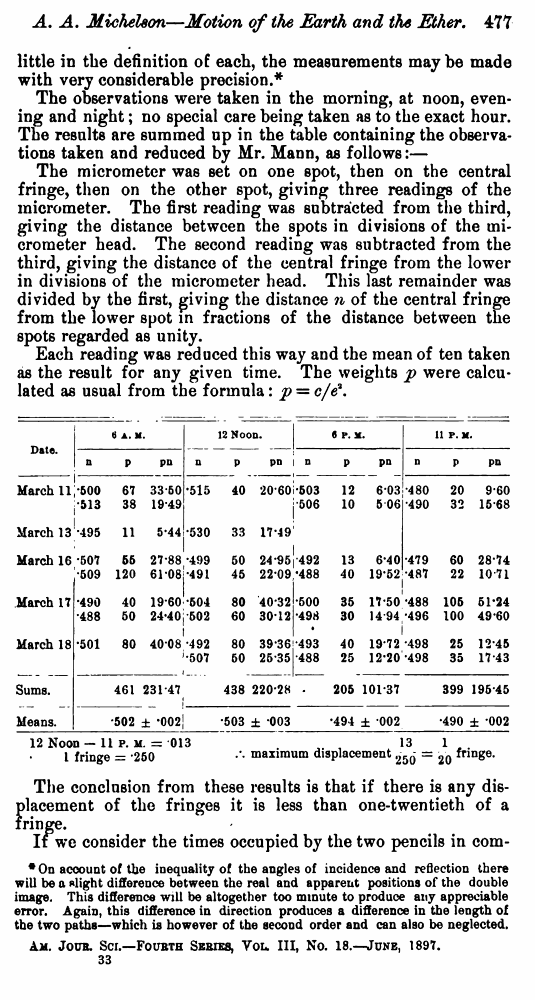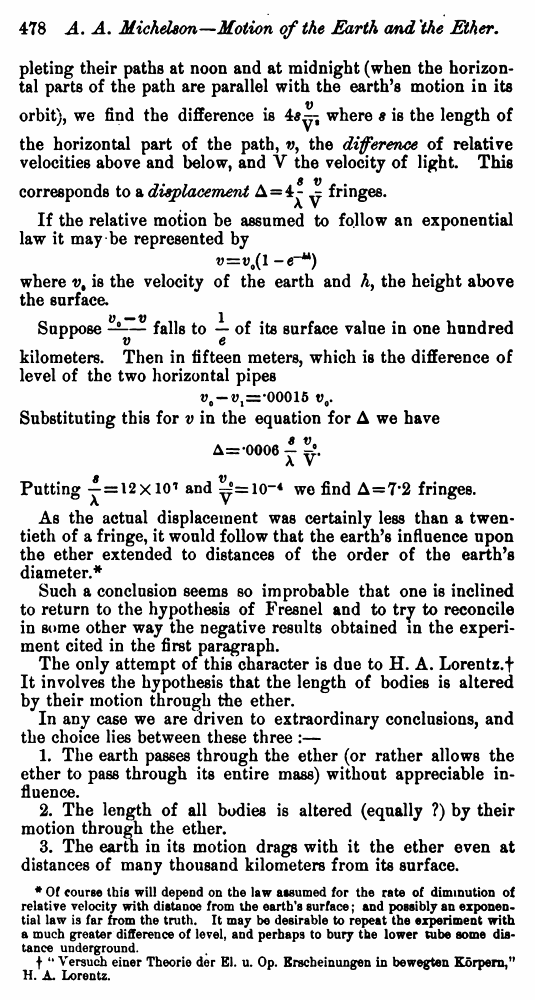Michelson A.A. The relative Motion of the Earth and the Ether. // American Journal of Science.—Fourth Series, Vol. III, No. 18.—June, 1897.

В начало   Другие форматы   <<<     Страница 477   >>>1  475  476  477 478

little in the definition of each, the measurements may be made with very considerable precision.*

The observations were taken in the morning, at noon, evening and night; no special care being taken as to the exact hour. The results are summed up in the table containing the observations taken and reduced by Mr. Mann, as follows:—

The micrometer was set on one spot, then on the central fringe, then on the other spot, giving three readings of the micrometer. The first reading was subtracted from the third, giving the distance between the spots in divisions of the micrometer head. The second reading was subtracted from the third, giving the distance of the central fringe from the lower in divisions of the micrometer head. This last remainder was divided by the first, giving the distance n of the central fringe from the lower spot in fractions of the distance between the spots regarded as unity.

Each reading was reduced this way and the mean of ten taken as the result for any given time. The weights p were calculated as usual from the formula: p = c/e2.

 Date. 6 A. M. 12 Noon. 6 P. M. 11 P. M. n P pn n P pn n P pn n P pn March 11 0.500 67 33.50 0.515 40 20.60 0.503 12 6.03 0.480 20 9.60 0.513 38 19.49 0.506 10 5.06 0.490 32 15.68 March 13 0.495 11 5.44 0.530 33 17.49 March 16 0.507 55 27.88 0.499 50 24.95 0.492 13 6.40 0.479 60 28.74 0.509 120 61.08 0.491 45 22.09 0.488 40 19.52 0.487 22 10.71 March 17 0.490 40 19.60 0.504 80 40.32 0.500 35 17.50 0.488 105 51.24 0.488 50 24.40 0.502 60 30.12 0.498 30 14.94 0.496 100 49.60 March 18 0.501 80 40.08 0.492 80 39.36 0.493 40 19.72 0.498 25 12.45 0.507 50 25.35 0.488 25 12.20 0.498 35 17.43 Sums. 461 231.47 438 220.28 205 101.37 399 195.45 Means. 0.502 ± 0.002 0.503 ± 0.003 0.494 ± 0.002 0.490 ± 0.002

12 Noon – 11 p. m. = 0.013

1 fringe = 0.250 ∴maximum displacement 13/250 = 1/20 fringe.

The conclusion from these results is that if there is any displacement of the fringes it is less than one-twentieth of a fringe.

If we consider the times occupied by the two pencils in com

* On account of the inequality of the angles of incidence and reflection there will be a slight difference between the real and apparent positions of the double image. This difference will be altogether too minute to produce any appreciable error. Again, this difference in direction produces a difference in the length of the two paths—which is however of the second order and can also be neglected.

Am. Joint. Sci.—Fourth Series, You III, No. 18.—June, 1897.

pleting their paths at noon and at midnight (when the horizontal parts of the path are parallel with the earth’s motion in its

orbit), we find the difference iswhere s is the length of

the horizontal part of the path, v, the difference of relative velocities above and below, and V the velocity of light. This

corresponds to a displacementfringes.

If the relative motion be assumed to follow an exponential law it may be represented by

where v, is the velocity of the earth and h, the height above the surface.

Supposefalls toof its surface value in one hundred

kilometers. Then in fifteen meters, which is the difference of level of the two horizontal pipes

Substituting this for v in the equation for Δ we have

Puttingandwe find Δ=7.2 fringes.

As the actual displacement was certainly less than a twentieth of a fringe, it would follow that the earth’s influence upon the ether extended to distances of the order of the earth’s diameter.*

Such a conclusion seems so improbable that one is inclined to return to the hypothesis of Fresnel and to try to reconcile in some other way the negative results obtained in the experiment cited in the first paragraph.

The only attempt of this character is due to H. A. Lorentz.† It involves the hypothesis that the length of bodies is altered by their motion through the ether.

In any case we are driven to extraordinary conclusions, and the choice lies between these three :—

1. The earth passes through the ether (or rather allows the ether to pass through its entire mass) without appreciable influence.

2. The length of all bodies is altered (equally ?) by their motion through the ether.

3. The earth in its motion drags with it the ether even at distances of many thousand kilometers from its surface.

* Of course this will depend on the law assumed for the rate of diminution of relative velocity with distanoe from the earth's surface; and possibly an exponential law is far from the truth. It may be desirable to repeat the experiment with a much greater difference of level, and perhaps to bury the lower tube some distance underground.

† "Versuch einer Theorie der El. u. Op. Erecheinungen in bewegten Körpern,” H. A. Lorentz.